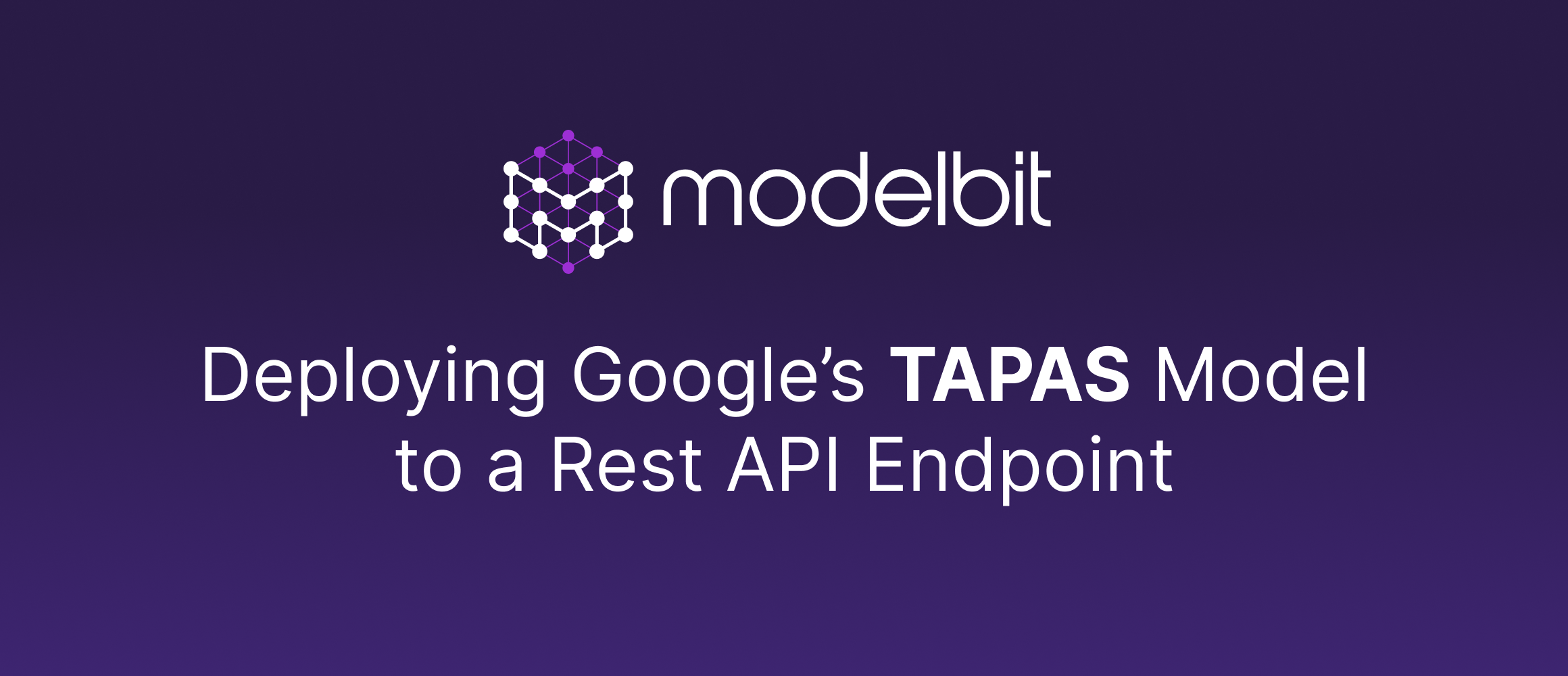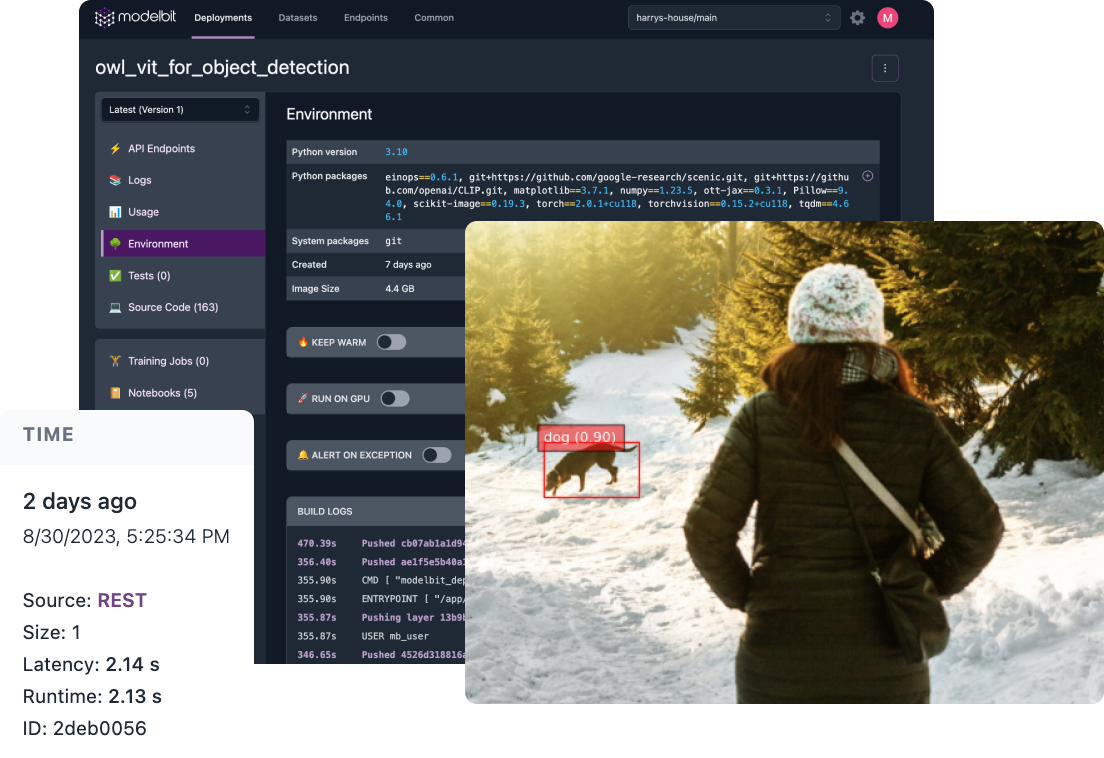# Deploying Google’s Table Q&A Model (TAPAS) to a REST API

Michael ButlerOops! Something went wrong while submitting the form.

## Introduction

In natural language research, Table Question Answering (Table QA) refers to models that can use tabular data to answer a user’s question.

For example, consider the following table:

Styled Table
Repository Stars Contributors Programming Language
Transformers 36542 651 Python
Datasets 4512 77 Rust
Tokenizers 3934 34 NodeJS

A user may want to ask questions of this data using only their natural language, as opposed to writing queries with code (SQL, Python) or selecting from a limited set of answers provided by an application.

Here are some questions we could ask based on this tabular data. Notice that the questions are increasingly complex and may include the need for aggregation:

• How many stars does the Transformers repository have?
• What is the sum of stars for the Datasets and Tokenizers repositories?
• Which programming languages are associated with repositories that have less than 5000 stars?

TAPAS (an acronym for TAble PArSing) is a BERT-based model from Google that can answer questions like these, and more, with impressive accuracy (see the benchmarks and research paper here).

In this blog post, we will show you how to deploy the TAPAS model into production with Modelbit. Once deployed, you can easily hand the appropriate REST API call to your engineers in order to incorporate the TAPAS model’s inferences into your web application or other production environment.

## Local setup in the notebook

Let us begin by looking at how to use TAPAS locally for making inferences. Open up any Python notebook and run the following code. Alternatively, you may use this project in Deepnote.

While the official TAPAS repository contains helpful demo notebooks, not all relevant setup instructions are documented. Instead, we can use the transformers model contributed by nielsr.

### Installation

``````
!pip install transformers==4.34.0
``````

### Imports

``````
import modelbit
from transformers import TapasTokenizer, TapasConfig, TapasForQuestionAnswering
import pandas as pd
from typing import Union
``````

TAPAS has been fine tuned on different datasets and offers several pre-trained models. Here, we are selecting the "tapas-large-finetuned-wikisql-supervised" model which responds well to queries involving aggregation.

``````
config = TapasConfig(model_name)
tokenizer = TapasTokenizer.from_pretrained(model_name)
``````

### Return an inference locally

We can use the code below (modified from code on the HuggingFace site) to return an inference locally within our notebook. The function below expects two inputs:

1. Data in the form of a dictionary
2. A string or list representing one or multiple questions
``````
def return_inference(data: dict, queries: Union[str, list]) -> dict:
table = pd.DataFrame.from_dict(data)
queries = [queries] if isinstance(queries, str) else queries
inputs = tokenizer(
)
outputs = model(**inputs)
(
predicted_aggregation_indices,
) = tokenizer.convert_logits_to_predictions(
inputs, outputs.logits.detach(), outputs.logits_aggregation.detach()
)

# let's print out the results:
id2aggregation = {0: "NONE", 1: "SUM", 2: "AVERAGE", 3: "COUNT"}
aggregation_predictions_string = [
id2aggregation[x] for x in predicted_aggregation_indices
]

if len(coordinates) == 1:
# only a single cell:
else:
# multiple cells
cell_values = []
for coordinate in coordinates:
cell_values.append(table.iat[coordinate])

results = {}
for query, answer, predicted_agg in zip(
):
)

return results

``````

### Define the dataset and questions

Let us use the example dataset and questions referenced at the beginning of the tutorial. In practice, you would likely be pulling data from your warehouse for development purposes.

``````
data = {
"Repository": ["Transformers", "Datasets", "Tokenizers"],
"Stars": ["36542", "4512", "3934"],
"Contributors": ["651", "77", "34"],
"Programming language": ["Python", "Rust", "NodeJS"],
}

queries = [
"How many stars does the transformers repository have?",
"what is the sum of stars for the Datasets and Tokenizers repositories?",
("Which programming languages are associated with " +
"repositories that have less than 5000 stars?"),
]
``````

### Call the "return_inference" function locally

``````
return_inference(data, queries)
``````

As you can see below, the output of the function lists all queries and their respective answers as provided by the TAPAS model.

``````
{
"How many stars does the transformers repository have?":
"COUNT of 36542",
"what is the sum of stars for the Datasets and Tokenizers repositories?":
"SUM of 4512, 3934",
"Which programming languages are associated with repositories that have less than 5000 stars?":
"Rust, NodeJS",
}
``````

## Deploy to production with Modelbit

Deploying to Modelbit is straightforward and requires two steps:

1. Connect the notebook to Modelbit
2. Deploy the "return_inference" function to Modelbit

### Connect the notebook to Modelbit

Simply run the code below to log in and connect the notebook to Modelbit.

``````
import modelbit
``````

You will be prompted with a URL that directs you to Modelbit to establish the connection to the notebook.

### Deploy the "return_inference" function to Modelbit

The last step is to deploy our "return_inference" function to Modelbit. This is where the actual deployment happens. Modelbit will package up all your source code and dependencies and make the model available via a REST API.

``````
mb.deploy(return_inference)
``````

After a successful deployment from the notebook, you can view the deployment details by clicking “View in Modelbit” or by visiting your Modelbit dashboard.

Once inside our Modelbit dashboard, we can see that Modelbit has created a containerized production environment that maintains all of our package dependencies.

Environment and dependencies created in Modelbit

The source code for the "return_inference" function is also available here.

## Call the model in production

Modelbit gives us a REST API to the model (as well as SQL APIs). We can simply open up a terminal and paste in the provided "curl" command to return results from the productionized model.

For example, here are the same inferences as before, but returned by directly calling the model in production. As you can see, we have the same results as the locally developed example above.

``````
import requests

url = "https://allancampopiano.app.modelbit.com/v1/tapas_inference/latest"

modelbit_data = {"data": [data, queries]}
r = requests.post(url, json=modelbit_data)
r.json()
``````
``````
{
"data": {
"How many stars does the transformers repository have?":
"COUNT of 36542",
"what is the sum of stars for the Datasets and Tokenizers repositories?":
"SUM of 4512, 3934",
"Which programming languages are associated with repositories that have less than 5000 stars?":
"Rust, NodeJS",
}
}
``````

## Next Steps

Modelbit is a fast, easy way to deploy any custom ML model to a REST Endpoint. We believe that ML teams who want to move fast need a modern alternative to SageMaker. Sign up for the free trial today to deploy your first ML model into production in minutes with just a few lines of code in any data science notebook.## Deploy Custom ML Models to Production with Modelbit

Join other world class machine learning teams deploying customized machine learning models to REST Endpoints.